# Test Prep Plan - Take a practice test

Take this practice test to check your existing knowledge of the course material. We'll review your answers and create a Test Prep Plan for you based on your results.
How Test Prep Plans work
1
2Based on your results, we'll create a customized Test Prep Plan just for you!
3Study smarter
Study more effectively: skip concepts you already know and focus on what you still need to learn.

# Praxis I Math: Right Triangles and Trigonometry Chapter Exam

Exam Instructions:

Choose your answers to the questions and click 'Next' to see the next set of questions. You can skip questions if you would like and come back to them later with the "Go To First Skipped Question" button. When you have completed the practice exam, a green submit button will appear. Click it to see your results. Good luck!

### Page 1

#### Question 1 1. What is x?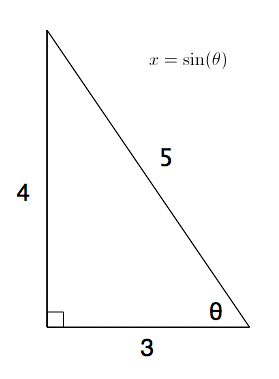#### Question 3 3. Find the shortest distance between a and b by drawing a line connecting them and using the Pythagorean Theorem.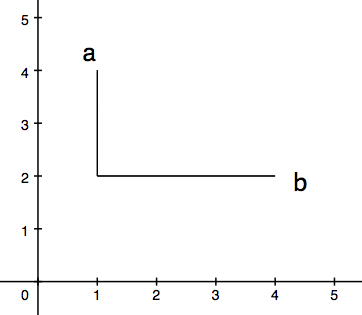#### Question 4 4. Simplify the following expression.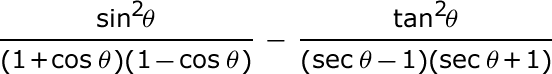#### Question 5 5. What is z in terms of x and y?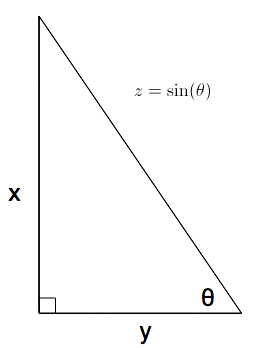### Page 2

#### Question 6 6. Jerry wants to buy a TV online, but the ad for the TV he wants to buy does not say what the screen size is. He knows that TV screens are measured diagonally. This image shows the length and width of the screen, so he decides to use the Pythagorean Theorem to determine the diagonal screen size. What is the screen size of the TV that Jerry wants to buy (to the nearest inch)?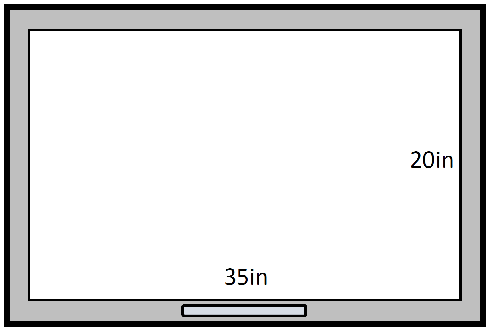#### Question 7 7. What's the value of 2sin(θ)cos(θ) given that the equation below is true?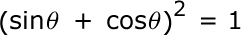#### Question 8 8. Solve the following trigonometric equation for x.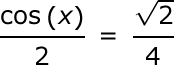#### Question 9 9. Simplify the following expression.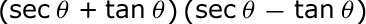#### Question 10 10. Solve the following equation for θ.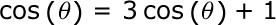### Page 3

#### Question 11 11. Solve for the value of x in the trigonometric equation below. (Use the domain -π/2 to π/2)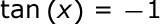#### Question 12 12. Solve the following trigonometric equation for θ.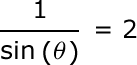#### Question 13 13. The lines a and b are parallel lines of length 5 and 2. The lines d and e are parallel to one another, and both are perpendicular to a and b. What is the length of c?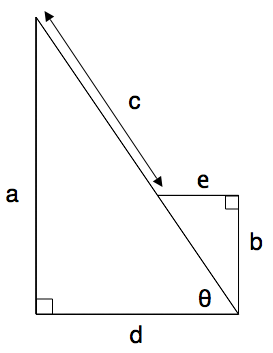#### Question 15 15. What is x?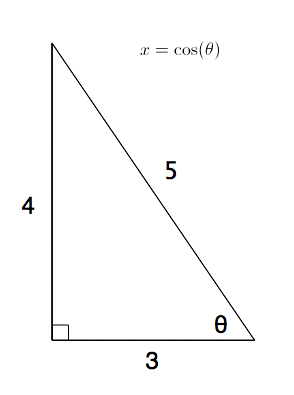### Page 4

#### Question 17 17. What is the value of cos(θ) given that the equation below is true?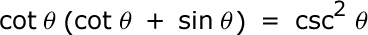#### Question 20 20. What is x?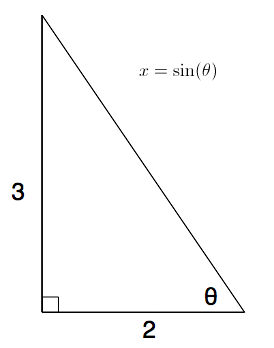### Page 5

#### Question 21 21. Which of the following is true?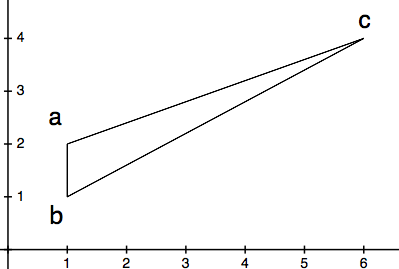#### Question 22 22. What is x?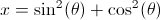#### Question 23 23. What is z in terms of x?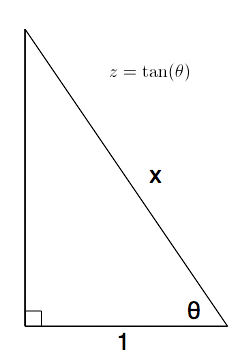### Page 6

#### Question 27 27. Solve the following equation for x.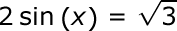#### Question 29 29. Which of these angles can replace x in tan (x)?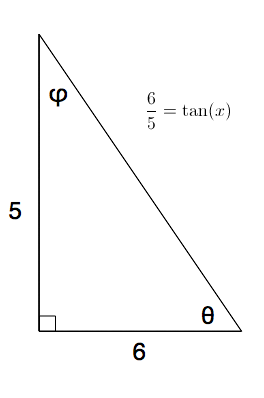#### Question 30 30. The triangle below has an altitude of 10. The longer split of the hypotenuse is 12. What is the measurement of the shorter split of the hypotenuse?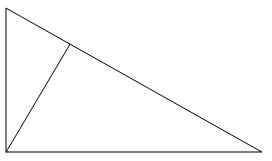#### Praxis I Math: Right Triangles and Trigonometry Chapter Exam Instructions

Choose your answers to the questions and click 'Next' to see the next set of questions. You can skip questions if you would like and come back to them later with the "Go To First Skipped Question" button. When you have completed the practice exam, a green submit button will appear. Click it to see your results. Good luck!

Support